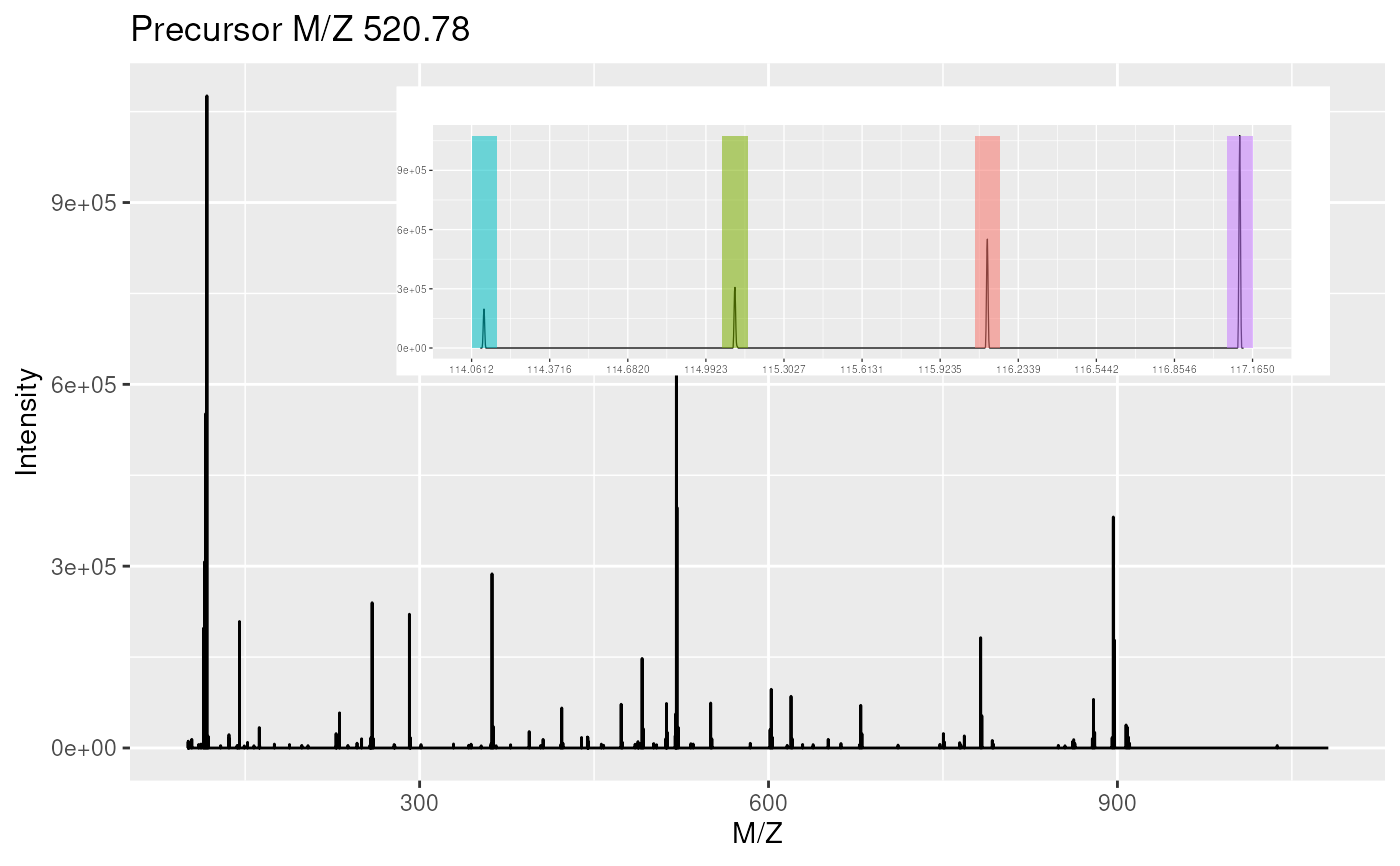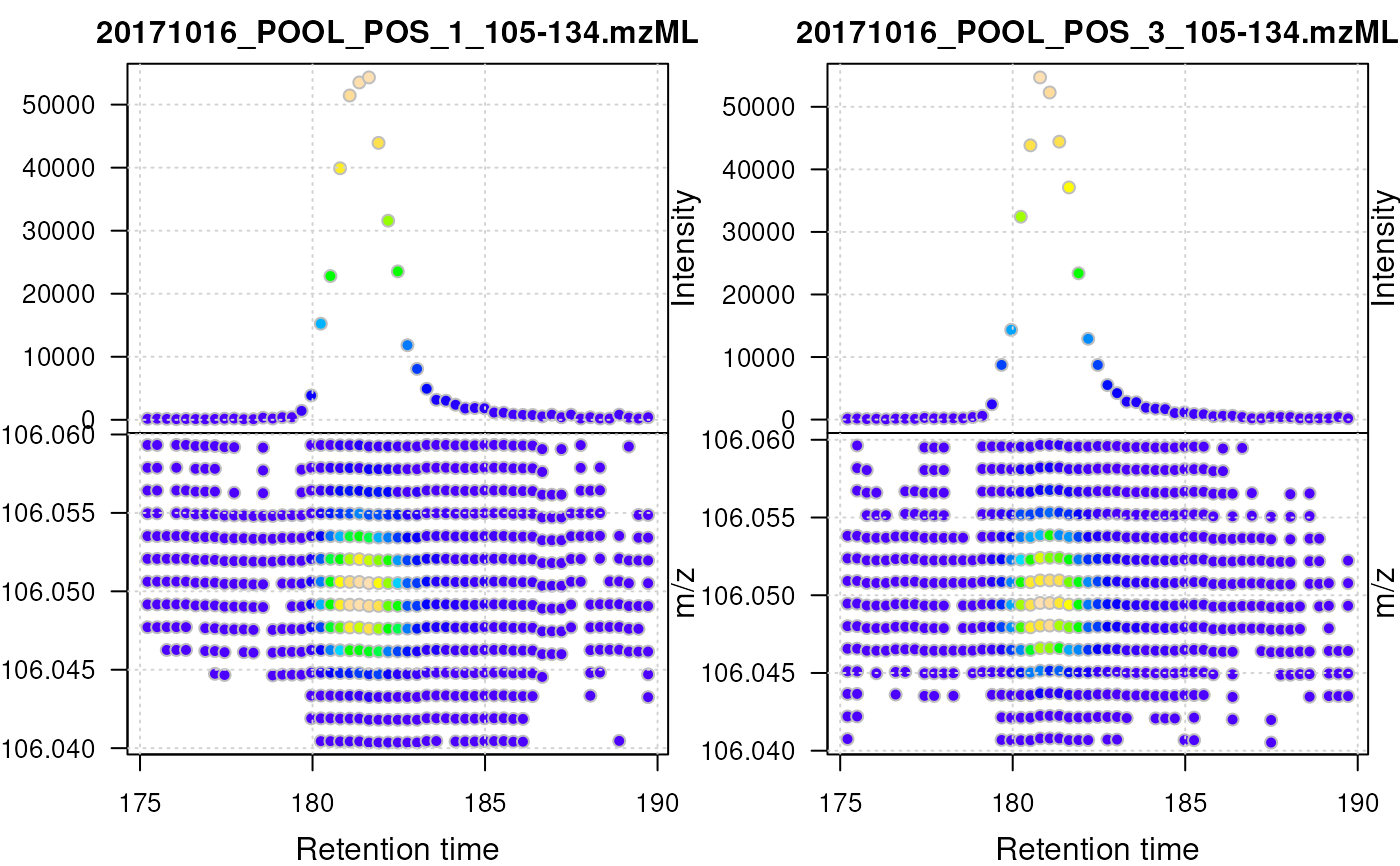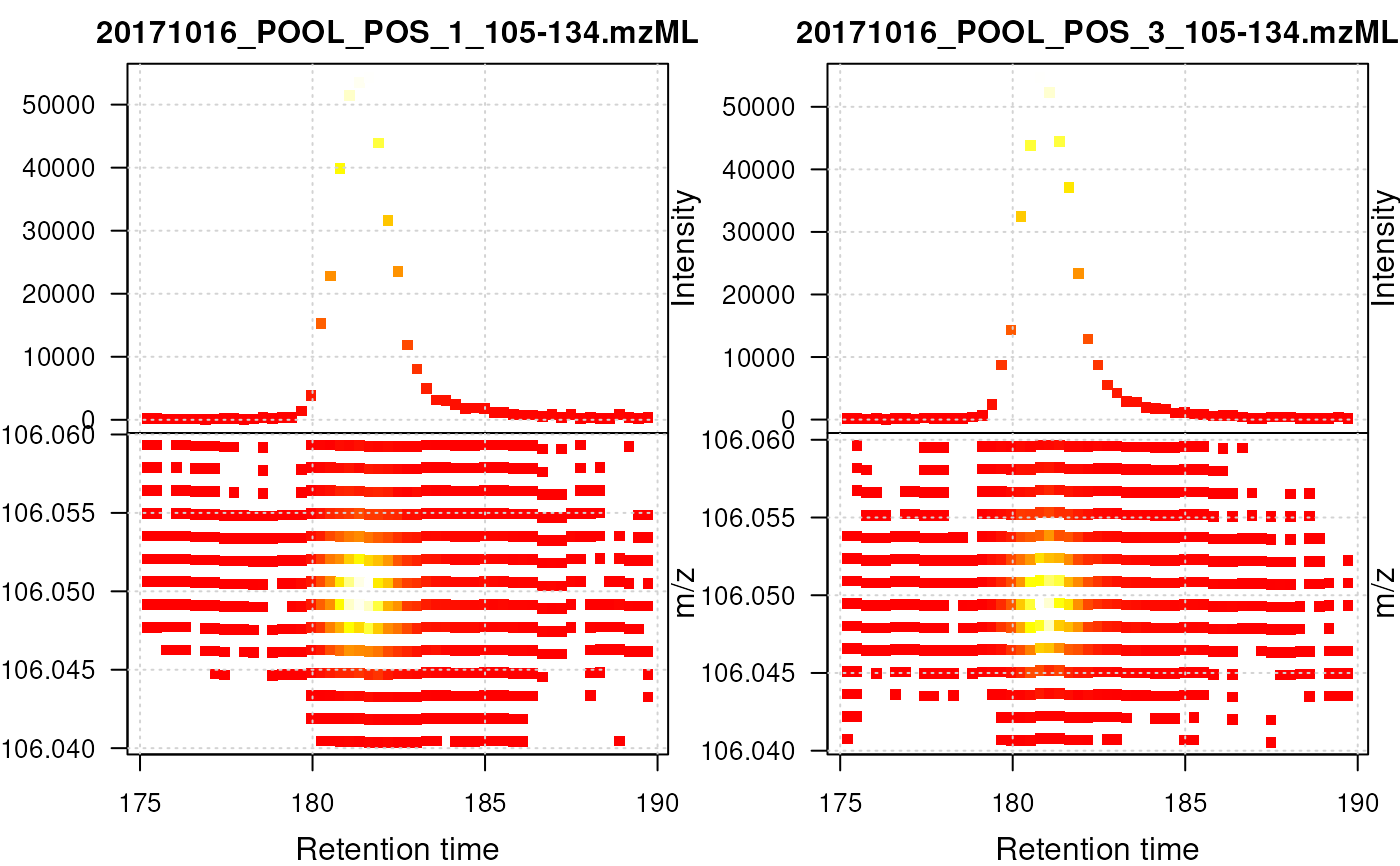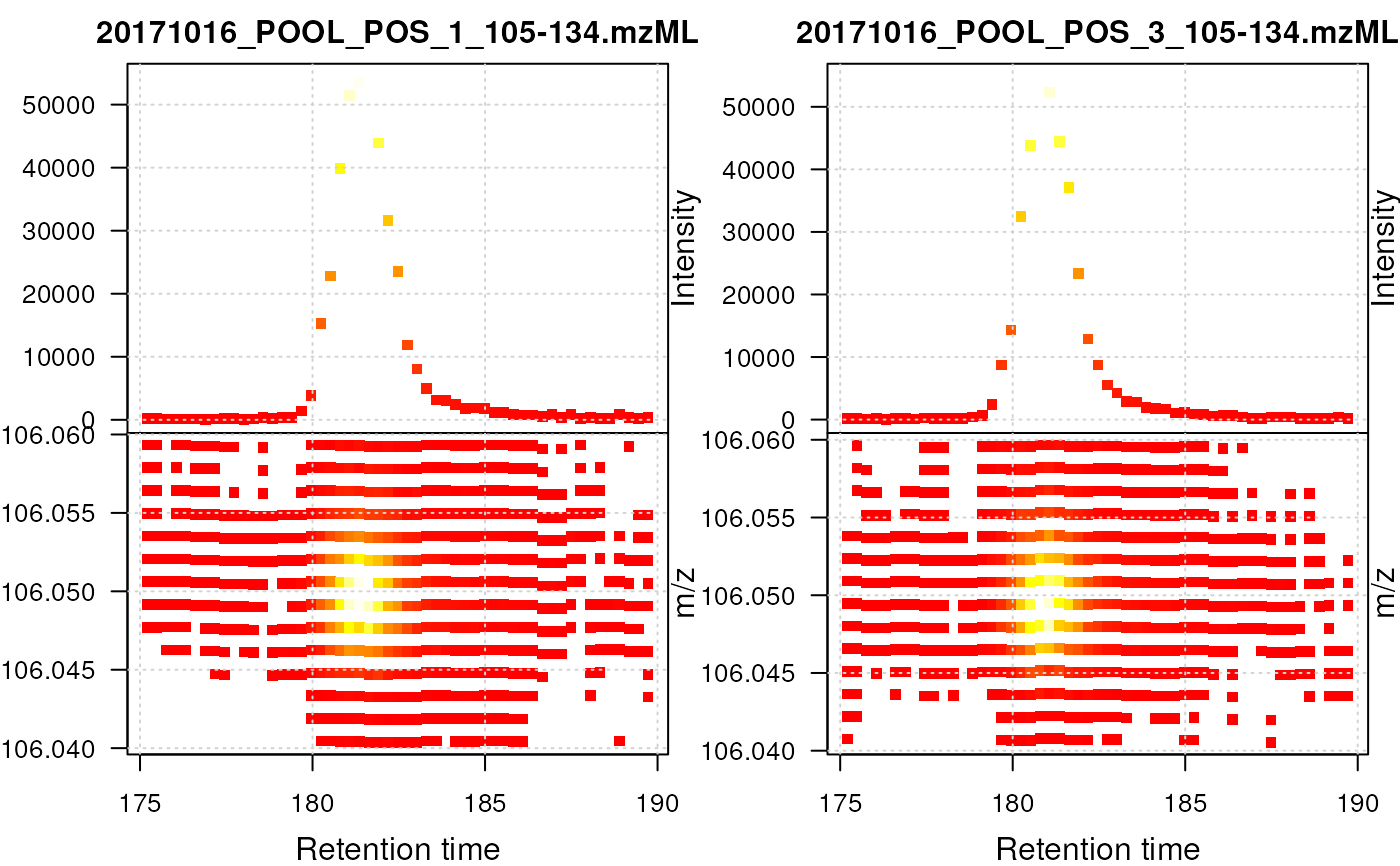These methods provide the functionality to plot mass spectrometry data provided as MSnExp, OnDiskMSnExp or Spectrum objects. Most functions plot mass spectra M/Z values against intensities.

Full spectra (using the full parameter) or specific peaks of interest can be plotted using the reporters parameter. If reporters are specified and full is set to 'TRUE', a sub-figure of the reporter ions is inlaid inside the full spectrum.

If an "MSnExp" is provided as argument, all the spectra are aligned vertically. Experiments can be subset to extract spectra of interest using the [ operator or extractPrecSpectra methods.

Most methods make use the ggplot2 system in which case an object of class 'ggplot' is returned invisibly.

If a single "Spectrum2" and a "character" representing a valid peptide sequence are passed as argument, the expected fragement ions are calculated and matched/annotated on the spectum plot.

Arguments

x Objects of class "Spectrum", "Spectrum2" or "MSnExp" to be plotted. Missing, "Spectrum" or "character". An object of class "ReporterIons" that defines the peaks to be plotted. If not specified, full must be set to 'TRUE'. Logical indicating whether full spectrum (respectively spectra) of only reporter ions of interest should be plotted. Default is 'FALSE', in which case reporters must be defined. Logical indicating if spectrum or spectra are in centroided mode, in which case peaks are plotted as histograms, rather than curves. Logical specifying whether plot should be printed to current device. Default is 'TRUE'. Width of sticks for full centroided spectra. Default is to use maximum MZ value divided by 500. Width of histogram bars for centroided reporter ions plots. Default is 0.01.

Methods

plot(signature(x = "MSnExp", y = "missing"), type = c("spectra", "XIC"), reporters = "ReporterIons", full = "logical", plot = "logical", ...)

For type = "spectra": Plots all the spectra in the MSnExp object vertically. One of reporters must be defined or full set to 'TRUE'. In case of MSnExp objects, repoter ions are not inlaid when full is 'TRUE'. For type = "XIC": Plots a combined plot of retention time against m/z values and retention time against largest signal per spectrum for each file. Data points are colored by intensity. The lower part of the plot represents the location of the individual signals in the retention time - m/z space, the upper part the base peak chromatogram of the data (i.e. the largest signal for each spectrum). This plot type is restricted to MS level 1 data and is most useful for LC-MS data. Ideally, the MSnExp (or OnDiskMSnExp) object should be filtered first using the filterRt and filterMz functions to narrow on an ion of interest. See examples below. This plot uses base R plotting. Additional arguments to the plot function can be passed with .... Additional arguments for type = "XIC" are:

col

color for the border of the points. Defaults to col = "grey".

colramp

color function/ramp to be used for the intensity-dependent background color of data points. Defaults to colramp = topo.colors.

grid.color

color for the grid lines. Defaults to grid.color = "lightgrey"; use grid.color = NA to disable grid lines altogether.

pch

point character. Defaults to pch = 21

.
...

additional parameters for the low-level plot function.

plot(signature(x = "Spectrum", y = "missing"), reporters = "ReporterIons", full = "logical", centroided. = "logical", plot = "logical", w1, w2)

Displays the MZs against intensities of the Spectrum object as a line plot. At least one of reporters being defined or full set to 'TRUE' is required. reporters and full are used only for "Spectrum2" objects. Full "Spectrum1" spectra are plotted by default.

plot(signature(x = "Spectrum2", y = "character"), orientation = "numeric", add = "logical", col = "character", pch, xlab = "character", ylab = "character", xlim = "numeric", ylim = "numeric", tolerance = "numeric", relative = "logical", type = "character", modifications = "numeric", x = "numeric", fragments = "data.frame", fragments.cex = "numeric", ... )

Plots a single MS2 spectrum and annotates the fragment ions based on the matching between the peaks in x and the fragment peaks calculated from the peptide sequence y. The default values are orientation=1, add=FALSE, col="#74ADD1", pch=NA, xlab="m/z", ylab="intensity", ylim=c(0, 1), tolerance=25e-6, relative=TRUE, type=c("b", "y"), modifications=c(C=160.030649), z=1, fragments=MSnbase:::calculateFragments_Spectrum2 and fragments.cex=0.75. Additional arguments ... are passed to plot.default.

calculateFragments to calculate ions produced by fragmentation and plot.Spectrum.Spectrum to plot and compare 2 spectra and their shared peaks.

Chromatogram for plotting of chromatographic data.

Author

Laurent Gatto <lg390@cam.ac.uk>, Johannes Rainer and Sebastian Gibb

Examples

data(itraqdata)
## plotting experiments
plot(itraqdata[1:2], reporters = iTRAQ4)plot(itraqdata[1:2], full = TRUE)
## plotting spectra
plot(itraqdata[],reporters = iTRAQ4, full = TRUE)itraqdata2 <- pickPeaks(itraqdata)
i <- 14
s <- as.character(fData(itraqdata2)[i, "PeptideSequence"])
plot(itraqdata2[[i]], s, main = s)